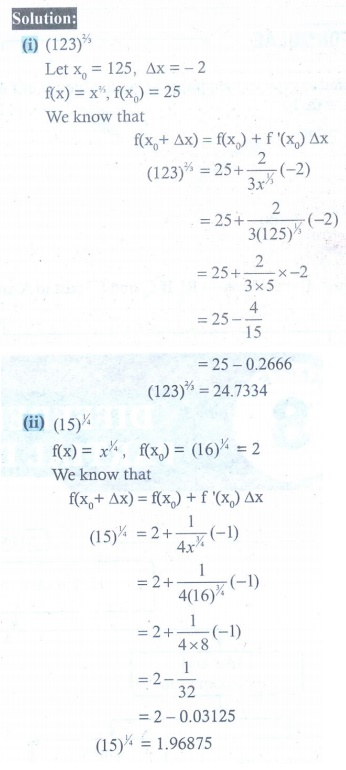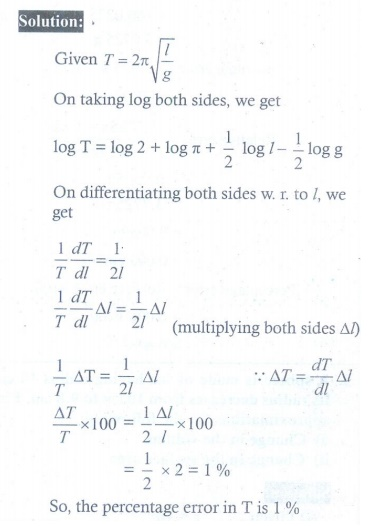Home | | Maths 12th Std | Exercise 8.1: Linear Approximation

# Exercise 8.1: Linear Approximation

Maths Book back answers and solution for Exercise questions - Mathematics : Differentials and Partial Derivatives: Linear Approximation, Errors: Absolute Error, Relative Error, and Percentage Error - Exercise Problem Questions with Answer, Solution

EXERCISE 8.1

1. Let f (x) = 3ŌłÜx. Find the linear approximation at x = 27 . Use the linear approximation to approximate 3ŌłÜ27.2 .2. Use the linear approximation to find approximate values of

(i) (123)2/3

(ii) 4ŌłÜ15

(iii) 3ŌłÜ263. Find a linear approximation for the following functions at the indicated points.

(i) f (x) = x3 ŌłÆ 5x + 12, x0 = 2

(ii) g ( x) = ŌłÜ[x2 + 9], x0 = ŌłÆ4

(iii) h(x) = x / x+1 , x0 = 14. The radius of a circular plate is measured as 12.65 cm instead of the actual length 12.5 cm. find the following in calculating the area of the circular plate:

(i) Absolute error (ii) Relative error (iii) Percentage error5. A sphere is made of ice having radius 10 cm. Its radius decreases from 10 cm to 9.8 cm. Find approximations for the following:

(i) change in the volume (ii) change in the surface area6. The time T , taken for a complete oscillation of a single pendulum with length , is given by the equation T = 2ŽĆŌłÜ(l/g) , where g is a constant. Find the approximate percentage error in the calculated value of T corresponding to an error of 2 percent in the value of l .7. Show that the percentage error in the nth root of a number is approximately 1/n times the percentage error in the number1. (i) 3.0074

2. (i) 24.73ŌĆā (ii) 1.9688ŌĆā (iii) 2.963

3. (i) 7x ŌłÆ 4 (ii) 9ŌłÆ4x / 4 (iii) x+1 /4

4. (i) 0.0225ŽĆ cm2,  (ii) 0.006ŌĆēcm2 (iii) 0.6%

5. (i) Volume decreases by 80ŽĆ cm3 ŌĆā (ii) Surface area decreases by 16ŽĆ cm2

6.ŌĆé 1%

Tags : Problem Questions with Answer, Solution , 12th Maths : UNIT 8 : Differentials and Partial Derivatives
Study Material, Lecturing Notes, Assignment, Reference, Wiki description explanation, brief detail
12th Maths : UNIT 8 : Differentials and Partial Derivatives : Exercise 8.1: Linear Approximation | Problem Questions with Answer, Solution